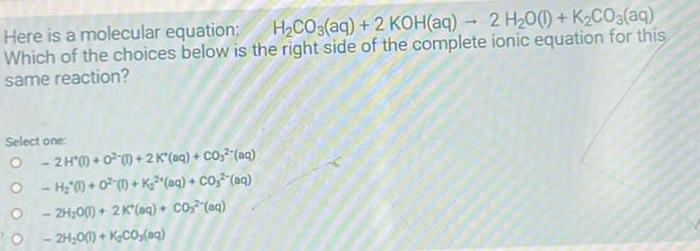Question:

# Here is a molecular equation: H₂CO3(aq) + 2 KOH(aq) → 2

Last updated: 8/9/2022Here is a molecular equation: H₂CO3(aq) + 2 KOH(aq) → 2 H₂O(l) + K₂CO3(aq) Which of the choices below is the right side of the complete ionic equation for this same reaction?